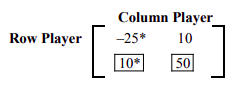# 11.1: Strictly Determined Games

$$\newcommand{\vecs}{\overset { \rightharpoonup} {\mathbf{#1}} }$$ $$\newcommand{\vecd}{\overset{-\!-\!\rightharpoonup}{\vphantom{a}\smash {#1}}}$$$$\newcommand{\id}{\mathrm{id}}$$ $$\newcommand{\Span}{\mathrm{span}}$$ $$\newcommand{\kernel}{\mathrm{null}\,}$$ $$\newcommand{\range}{\mathrm{range}\,}$$ $$\newcommand{\RealPart}{\mathrm{Re}}$$ $$\newcommand{\ImaginaryPart}{\mathrm{Im}}$$ $$\newcommand{\Argument}{\mathrm{Arg}}$$ $$\newcommand{\norm}{\| #1 \|}$$ $$\newcommand{\inner}{\langle #1, #2 \rangle}$$ $$\newcommand{\Span}{\mathrm{span}}$$ $$\newcommand{\id}{\mathrm{id}}$$ $$\newcommand{\Span}{\mathrm{span}}$$ $$\newcommand{\kernel}{\mathrm{null}\,}$$ $$\newcommand{\range}{\mathrm{range}\,}$$ $$\newcommand{\RealPart}{\mathrm{Re}}$$ $$\newcommand{\ImaginaryPart}{\mathrm{Im}}$$ $$\newcommand{\Argument}{\mathrm{Arg}}$$ $$\newcommand{\norm}{\| #1 \|}$$ $$\newcommand{\inner}{\langle #1, #2 \rangle}$$ $$\newcommand{\Span}{\mathrm{span}}$$$$\newcommand{\AA}{\unicode[.8,0]{x212B}}$$

In this chapter, we will study games that involve only two players. In these games, since a win for one person is a loss for the other, we refer to them as two-person zero-sum games. Although the games we will study here are fairly simple, they will provide us with an understanding of how games work and how they are applied in practical situations. We begin with an example.

## Example $$\PageIndex{1}$$

Robert and Carol decide to play a game using a dime and a quarter. Each chooses one of the two coins, puts it in their hand and closes their fist. At a given signal, they simultaneously open their fists. If the sum of the coins is less than 35 cents, Robert gets both coins, otherwise, Carol gets both coins. Write the matrix for the game, determine the optimal strategies for each player, and find the expected payoff for Robert.

Solution

Suppose Robert is the row player, that is, he plays the rows, and Carol is a column player. If Robert shows a dime and Carol shows a dime, the sum will be less than thirty five cents and Robert will win ten cents. But, if Robert shows a dime and Carol shows a quarter, the sum will not be less than thirty five cents and Carol will win ten cents or Robert will lose ten cents. The following matrix depicts all four cases and their corresponding payoffs for Robert. Remember a negative value is a loss for Robert and a win for Carol.The best strategy for Robert is to always show a dime because this way the worst he can do is lose ten cents. And, the best strategy for Carol is to always show a quarter because that way the worst she can do is to lose ten cents. If both Robert and Carol play their optimal strategies, Robert will lose ten cents each time. Therefore, the value of the game is negative ten cents.

In the above example, since there is only one fixed optimal strategy for each player, regardless of their opponent's strategy, we say the game possesses a pure strategy and is strictly determined.

Next, we formulate a method to find the optimal strategy for each player and the value of the game. The method involves considering the worst scenario for each player.

To consider the worst situation, the row player considers the minimum value in each row, and the column player considers the maximum value in each column. Note that the maximum value really represents a minimum value for the column player because the game matrix depicts the payoffs for the row player. We list the method below.

## Finding the Optimal Strategy and the Value for Strictly Determined Games

1. Put an asterisk(*) next to the minimum entry in each row.
2. Put a box around the maximum entry in each column.
3. The entry that has both an asterisk and a box represents the value of the game and is called a saddle point.
4. The row that is associated with the saddle point represents the best strategy for the row player, and the column that is associated with the saddle point represents the best strategy for the column player.
5. A game matrix can have more than one saddle point, but all saddle points have the same value.
6. If no saddle point exists, the game is not strictly determined. Non-strictly determined games are the subject of the next section.

## Example $$\PageIndex{2}$$

Find the saddle points and optimal strategies for the following game.Solution

We find the saddle point by placing an asterisk next to the minimum entry in each row, and by putting a box around the maximum entry in each column as shown below.Since the second row, first column entry, which happens to be 10, has both an asterisk and a box, it is a saddle point. This implies that the value of the game is 10, and the optimal strategy for the row player is to always play row 2, and the optimal strategy for the column player is to always play column 1. If both players play their optimal strategies, the row player will win 10 units each time.

The row player's strategy is written as $$\left[\begin{array}{ll} 0 & 1 \end{array}\right]$$ indicating that he will play row 1 with a probability of 0 and row 2 with a probability of 1.

Similarly the column player's strategy is written as $$\left[\begin{array}{l} 1 \\ 0 \end{array}\right]$$ implying that he will play column 1 with a probability of 1, and column 2 with a probability of 0.

This page titled 11.1: Strictly Determined Games is shared under a CC BY 4.0 license and was authored, remixed, and/or curated by Rupinder Sekhon and Roberta Bloom via source content that was edited to the style and standards of the LibreTexts platform; a detailed edit history is available upon request.